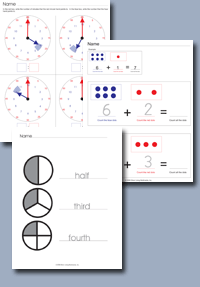﻿ Common Core Standards for Math - Kindergarten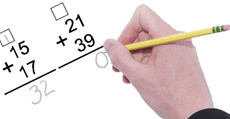Adaptive Worksheets

Helping Every Child to Succeed

toll-free (888) 777-0876
fax (888) 777-0875

Counting and Cardinality - Kindergarten

## Know number names and the count sequence

K.CC.1 - Count to 100 by ones and tens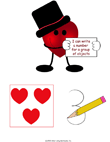"I Can" Posters with a Valentine's Day Theme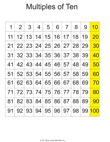100 Chart - Count by Tens This hundred chart can be used to teaching counting by 10 to 100.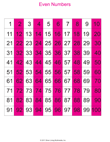Chart of Even Numbers Up to 100 Use this chart to teach even numbers and counting by ones and twos.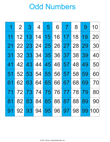Chart of Odd Numbers Up to 100 Use this chart to teach even numbers and counting by ones and twos.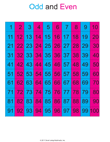100 Chart This hundred chart has even and odd numbers highlighted.100 Chart This hundred chart has multiples of 5 and 10 highlighted.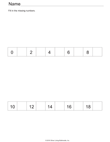Fill in the Missing Odd Numbers to 100 Set of 3 Worksheets.  Students must fill in the missing odd numbers up to 99.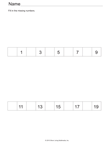Fill in the Missing Even Numbers to 100 Set of 3 Worksheets.  Students must fill in the missing even numbers up to 100.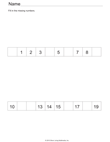Fill in Random Missing Numbers Up to 100 Set of 3 Worksheets.  Students must fill in the missing numbers up to 100.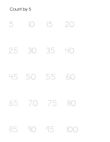Trace Multiples of 5 Up to 100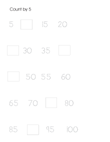Fill In Multiples of 5 Up to 100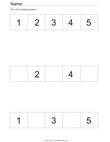Fill In the Missing Numbers Up to 10 Set of 3 Worksheets.   Students must fill in the missing numbers.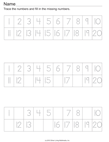Fill In the Missing Numbers Up to 20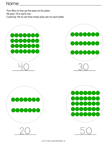Count By Tens

K.CC.2 - Count forward beginning from a given number within the known sequence (instead of having to begin at 1)

## K.CC.3 - Write numbers from 0 to 20. Represent a number of objects with a written numeral 0-20 (with 0 representing a count of no objects)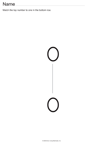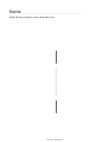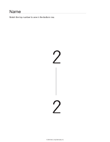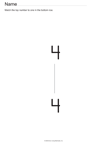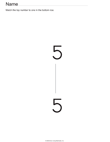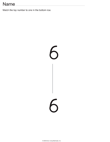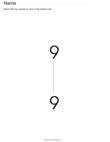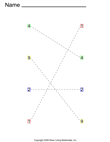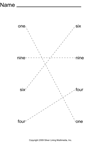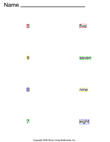Numeral to Numeral Matching Word to Word Matching Numeral to Word Matching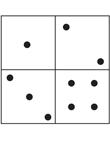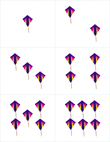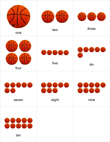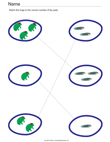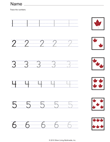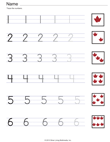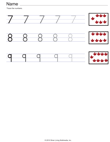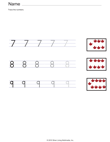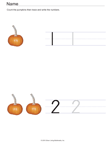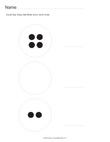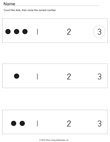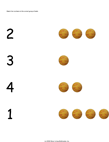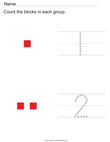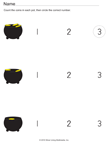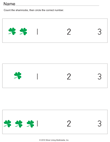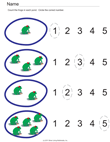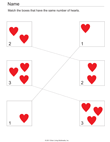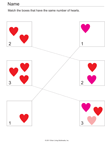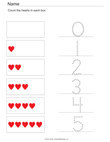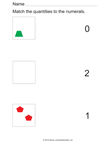## Count to tell the number of objects

K.CC.4  -Understand the relationship between numbers and quantities; connect counting to cardinality.

When counting objects, say the number names in the standard order, pairing each object with one and only one number name and each number name with one and only one object.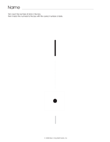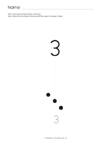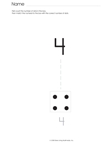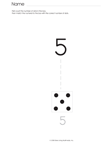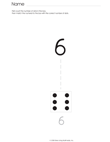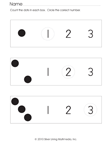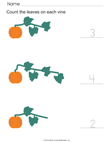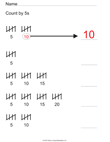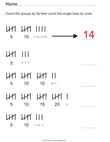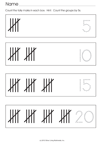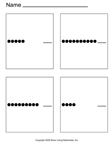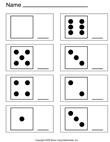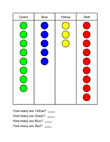Horizontal Counting Counting Dice Counting

Understand that the last number name said tells the number of objects counted. The number of objects is the same regardless of their arrangement or the order in which they were counted.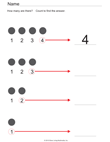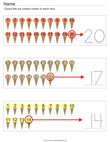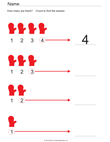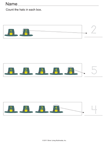Understand that each successive number name refers to a quantity that is one larger.

K.CC.5 - Count to answer “how many?” questions about as many as 20 things arranged in a line, a rectangular array, or a circle, or as many as 10 things in a scattered configuration; given a
number from 1–20, count out that many objects
.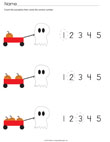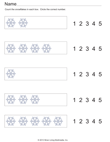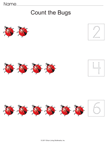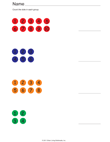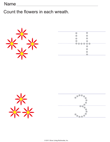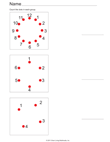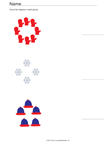Compare numbers

K.CC.6 - Identify whether the number of objects in one group is greater than, less than, or equal to the number of objects in another group, e.g., by using matching and counting strategies.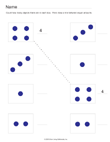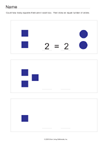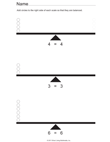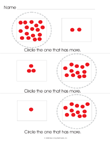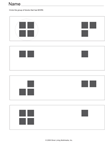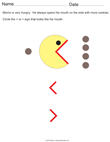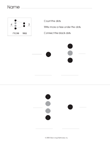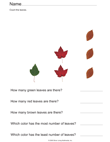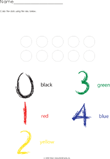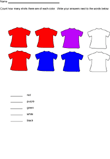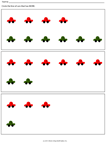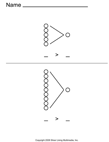Greater Than Less Than

K.CC.7 - Compare two numbers between 1 and 10 presented as written numerals.